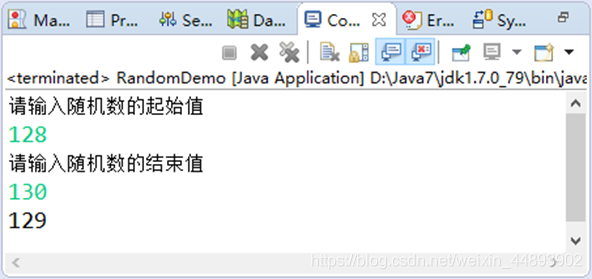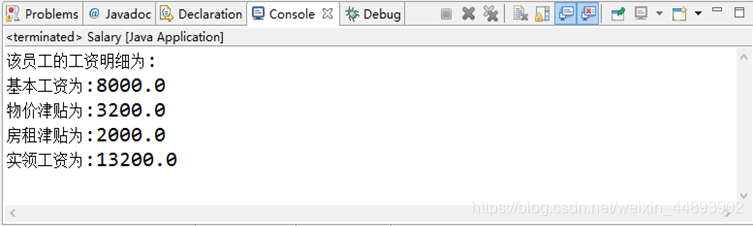+关注继续查看

# CUDA编程（二）

## CUDA初始化与核函数

### CUDA初始化#include <stdio.h>

//CUDA RunTime API
#include <cuda_runtime.h>

//CUDA 初始化
bool InitCUDA()
{
int count;

//取得支持Cuda的装置的数目
cudaGetDeviceCount(&count);

//没有符合的硬件
if (count == 0) {
fprintf(stderr, "There is no device.\n");
return false;
}

int i;

for (i = 0; i < count; i++) {
if (cudaGetDeviceProperties(&prop, i) == cudaSuccess) {
if (prop.major >= 1) {
break;
}
}
}

if (i == count) {
fprintf(stderr, "There is no device supporting CUDA 1.x.\n");
return false;
}

cudaSetDevice(i);

return true;
}

cudaGetDeviceProperties除了可以获得装置支持的 CUDA 版本之外，还有装置的名称、内存的大小、最大的 thread 数目、执行单元的频率等等。详情可参考NVIDIA 的 CUDA Programming Guide。

int main()
{

if (!InitCUDA())
{
return 0;
}

printf("CUDA initialized.\n"); return 0;

}#include <stdio.h>

//CUDA RunTime API
#include <cuda_runtime.h>

//CUDA 初始化
bool InitCUDA()
{
int count;

//取得支持Cuda的装置的数目
cudaGetDeviceCount(&count);

//没有符合的硬件
if (count == 0) {
fprintf(stderr, "There is no device.\n");
return false;
}

int i;

for (i = 0; i < count; i++) {
if (cudaGetDeviceProperties(&prop, i) == cudaSuccess) {
if (prop.major >= 1) {
break;
}
}
}

if (i == count) {
fprintf(stderr, "There is no device supporting CUDA 1.x.\n");
return false;
}

cudaSetDevice(i);

return true;
}

int main()
{

if (!InitCUDA())
{
return 0;
}

printf("CUDA initialized.\n"); return 0;

}


### CUDA核函数

#define DATA_SIZE 1048576

int data[DATA_SIZE];

//产生大量0-9之间的随机数
void GenerateNumbers(int *number, int size)
{
for (int i = 0; i < size; i++) {
number[i] = rand() % 10;
}
}

//生成随机数(main中调用)
//GenerateNumbers(data, DATA_SIZE);


#### Host&Device架构：//生成随机数
GenerateNumbers(data, DATA_SIZE);

/*把数据复制到显卡内存中*/

int* gpudata, *result;

//cudaMalloc 取得一块显卡内存 ( 其中result用来存储计算结果 )
cudaMalloc((void**)&gpudata, sizeof(int)* DATA_SIZE);
cudaMalloc((void**)&result, sizeof(int));

//cudaMemcpy 将产生的随机数复制到显卡内存中
//cudaMemcpyHostToDevice - 从内存复制到显卡内存
//cudaMemcpyDeviceToHost - 从显卡内存复制到内存
cudaMemcpy(gpudata, data, sizeof(int)* DATA_SIZE,cudaMemcpyHostToDevice);



#### CUDA核函数：

// __global__ 函数 (GPU上执行) 计算立方和
__global__ static void sumOfSquares(int *num, int* result)
{
int sum = 0;

int i;

for (i = 0; i < DATA_SIZE; i++) {

sum += num[i] * num[i] * num[i];

}

*result = sum;

}

#### 执行核函数：

    函数名称<<<block 数目, thread 数目, shared memory 大小>>>(参数...);

    sumOfSquares<<<1, 1, 0>>>(gpudata, result);

    int sum;

//cudaMemcpy 将结果从显存中复制回内存
cudaMemcpy(&sum, result, sizeof(int), cudaMemcpyDeviceToHost);

//Free
cudaFree(gpudata);
cudaFree(result);


    printf("GPUsum: %d \n", sum);

    sum = 0;

for (int i = 0; i < DATA_SIZE; i++) {
sum += data[i] * data[i] * data[i];
}

printf("CPUsum: %d \n", sum);



### 完整程序：

#### 程序代码：

#include <stdio.h>
#include <stdlib.h>

//CUDA RunTime API
#include <cuda_runtime.h>

#define DATA_SIZE 1048576

int data[DATA_SIZE];

//产生大量0-9之间的随机数
void GenerateNumbers(int *number, int size)
{
for (int i = 0; i < size; i++) {
number[i] = rand() % 10;
}
}

//CUDA 初始化
bool InitCUDA()
{
int count;

//取得支持Cuda的装置的数目
cudaGetDeviceCount(&count);

if (count == 0) {
fprintf(stderr, "There is no device.\n");
return false;
}

int i;

for (i = 0; i < count; i++) {
if (cudaGetDeviceProperties(&prop, i) == cudaSuccess) {
if (prop.major >= 1) {
break;
}
}
}

if (i == count) {
fprintf(stderr, "There is no device supporting CUDA 1.x.\n");
return false;
}

cudaSetDevice(i);

return true;
}

// __global__ 函数 (GPU上执行) 计算立方和
__global__ static void sumOfSquares(int *num, int* result)
{
int sum = 0;

int i;

for (i = 0; i < DATA_SIZE; i++) {

sum += num[i] * num[i] * num[i];

}

*result = sum;

}

int main()
{

//CUDA 初始化
if (!InitCUDA()) {
return 0;
}

//生成随机数
GenerateNumbers(data, DATA_SIZE);

/*把数据复制到显卡内存中*/

int* gpudata, *result;

//cudaMalloc 取得一块显卡内存 ( 其中result用来存储计算结果 )
cudaMalloc((void**)&gpudata, sizeof(int)* DATA_SIZE);
cudaMalloc((void**)&result, sizeof(int));

//cudaMemcpy 将产生的随机数复制到显卡内存中
//cudaMemcpyHostToDevice - 从内存复制到显卡内存
//cudaMemcpyDeviceToHost - 从显卡内存复制到内存
cudaMemcpy(gpudata, data, sizeof(int)* DATA_SIZE, cudaMemcpyHostToDevice);

// 在CUDA 中执行函数 语法：函数名称<<<block 数目, thread 数目, shared memory 大小>>>(参数...);
sumOfSquares << <1, 1, 0 >> >(gpudata, result);

/*把结果从显示芯片复制回主内存*/

int sum;

//cudaMemcpy 将结果从显存中复制回内存
cudaMemcpy(&sum, result, sizeof(int), cudaMemcpyDeviceToHost);

//Free
cudaFree(gpudata);
cudaFree(result);

printf("GPUsum: %d \n", sum);

sum = 0;

for (int i = 0; i < DATA_SIZE; i++) {
sum += data[i] * data[i] * data[i];
}

printf("CPUsum: %d \n", sum);

return 0;
}



#### 运行结果：### 总结：iOS的App模块化编程的框架实现方案——BeeHive
BeeHive是用于iOS的App模块化编程的框架实现方案，吸收了Spring框架Service的理念来实现模块间的API耦合。
319 0Java初学者作业——编写 Java 程序，让用户输入指定数字实现产生随机数。
Java初学者作业——编写 Java 程序，让用户输入指定数字实现产生随机数。
28 0Linux字符设备驱动总结程序（二）
1032 0Java初学者作业——编写程序计算实发工资（实践1）
Java初学者作业——编写程序计算实发工资（实践1）
53 0《Linux设备驱动开发详解 A》一一第３章Linux内核及内核编程
1329 0SCALA中的函数式编程
1078 0万事开头难，上篇已经起了一个头，之后的事情相对就简单了。上次定义了框架所需的dtd也就是规定了xml中该怎么写，有哪些元素。并且我们也让dtd和xml绑定在了一起，使dtd对xml的格式进行校验，并且在DocumentHolder中提供了根据xml文件路径获取xml文件的Document对象。
623 0IOS启用WebApp全屏模式
2140 01315 0
77

0